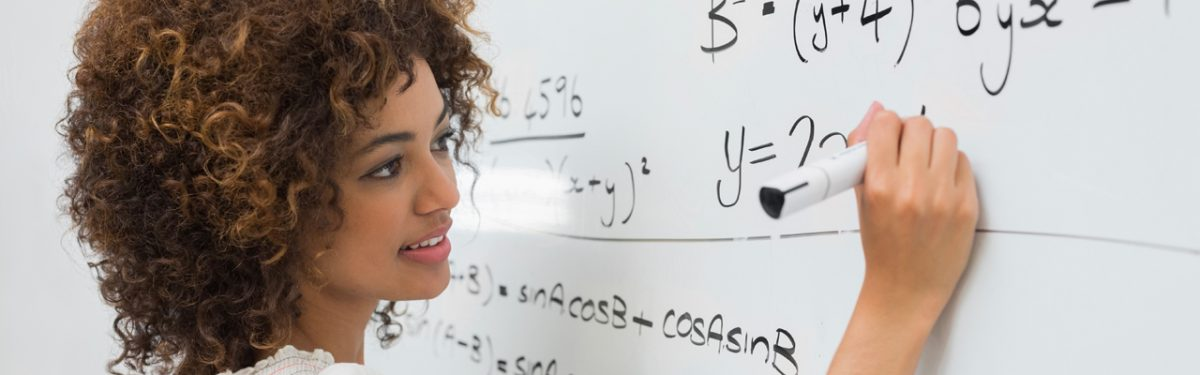# LINR 1 | Lesson 1 | Making Final Connections

## Making Final Connections

1. If you know a linear pattern is growing by 5, what other information would you need to determine the equation?

2. If you know a linear pattern has 10 squares in step 4, what other information would you need to determine the equation?

3. If you know a pattern has 42 squares in step 1 and grows by 3, what is the equation?

Check solutions here.